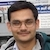# NAEST 2014 Screening Test: Equilibrium of Floating BodiesBy

A body float in a liquid if its density is less than that of the liquid. The net force on the floating body is zero i.e., it is in equilibrium. The equilibrium can be stable or unstable. We will explore the equilibrium of a body floating in a liquid.

Question 1: Guess the difference in the constructions of the pipes?

Question 2: With this difference why one floats vertically but the other falls on the surface?

Answer: Let a body of density $\rho_b$ and volume $V_b$ is floating in a liquid of density $\rho_l\, (>\rho_b)$. The forces acting on the body are its weight $W$ and buoyant force $F$ by the liquid i.e., \begin{align} W&=\rho_b V_b g\;\;\text{(downward)}, \\ F&=\rho_l V_l g\;\;\text{(upward)}, \end{align} where $V_l$ is the volume of the displaced liquid.

In equilibrium, the net force on the body is zero i.e., $W=F$, which gives \begin{align} \frac{V_l}{V_b}=\frac{\rho_b}{\rho_l} \end{align} If $\rho_b=\rho_l$ then $V_l=V_g$ i.e., the body is completely immersed in the liquid. If $\rho_b<\rho_l$ then the portion of the body floating out of the liquid is given by \begin{align} \frac{V_b-V_l}{V_b}=1-\frac{V_l}{V_b}=1-\frac{\rho_b}{\rho_l}. \end{align} If the liquid is water then $\rho_b/\rho_l=\rho$ is the specific gravity of the body. Thus, a body of specific gravity 0.8 floats in water with 20% of its volume outside the water.

The net force on a floating body is zero. What about the net torque? In equilibrium, the net torque is also zero. If a body is slightly disturbed from its equilibrium position then the torque on the body about its centre of mass becomes non-zero. This non-zero torque is called disturbance torque. If the disturbance torque brings back the body to its original position then the equilibrium is called a stable equilibrium otherwise it is called an unstable equilibrium. To know the disturbance torque, we should know the point where gravitational force and buoyant force acts.

We can assume gravitational force $W$ to act on the centre of mass Cb of the body. Similarly, the buoyant force $F$ acts on the centre of mass Cl of the displaced liquid. If Cb is below Cl then the body is in stable equilibrium. Thus, a cylinder with a heavy weight attached at its bottom floats in stable equilibrium. Let us analyse it with an example.

Let a cylinder AB has a non-uniform mass distribution such that its centre of mass Cb is close to the end A (see figure). Let length $h$ of the cylinder is immersed in the liquid. The centre of mass Cl of the displaced liquid is at the midpoint of the immersed part. The distance between Cb and Cl is $y$. The cylinder floats vertically with $W$ and $F$ lying in the same vertical line.Now, let us tilt the cylinder in clockwise direction by a small angle $\theta$. The forces on the cylinder remain the same but they no longer pass through the same vertical line. These forces form a couple of arm length $y\sin\theta\approx y\theta$. The couple produce an anticlockwise torque of magnitude $W y \theta$. This anticlockwise torque will try to bring back the cylinder in the initial vertical orientation. Thus, the equilibrium is stable. When disturbed, the cylinder will perform SHM. Can you find the time period of SHM?

Now, consider the other case in which Cb is close to end B (see figure). If cylinder is immersed vertically and there is no disturbance then it will float vertically with $W$ and $F$ lying in the same vertical line.Now, let us tilt the cylinder in clockwise direction by a small angle $\theta$. This time, the couple produces a clockwise torque of magnitude $W y \theta$. This clockwise torque will further tilt the cylinder. The equilibrium is unstable.